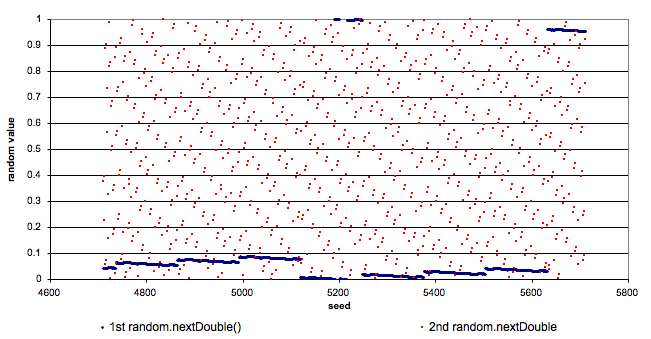# Java-related information

MATSim is written in Java. While Java is widely known, from time to time we stumble over certain features or specialities we’d like to highlight. This is the place to collect interesting and informative stuff about Java which might (or might not) have some relation to our code.

## Problems with HashMap, HashSet and Iterator

`HashSet` and `HashMap` do not specify in which order elements are iterated, possibly resulting in different results if the same code is run multiple times. This can lead to tests that randomly fail, further making debugging very hard. Use `LinkedHashSet/LinkedHashMap` instead. Those can be iterated over insertion order, leading to deterministic behavior.

## Random Numbers

### Introduction to random numbers

Our simulations depend heavily on random numbers. But despite using random numbers, we still want the simulations to be deterministic, so that results can be reproduced by running the same scenario a second time.

Usually, random numbers in Java are generated by calling `Math.random()`:

``````double d = Math.random();
``````

While this method returns a random number, the number is indeed random such as that in a second run of the same scenario, other random numbers would result and the simulation would no longer be deterministic. To overcome this problem, an instance of `java.util.Random` can be generated and initialized with a custom random seed:

``````Random random = new Random();
random.setSeed(1234);
...
double d = random.nextDouble();
``````

In this case, everytime the code is run, we get the same random numbers. As drawing random numbers is widely used in the code, MATSim offers a global instance of `Random`, which is automatically initialized with the seed specified in the configuration file:

``````import org.matsim.core.gbl.MatsimRandom;
...
double d = MatsimRandom.random.nextDouble();
``````

### Choosing a random seed

What value should be used as random seed? Is a value of `1` better than the value `86294`? As both numbers have the same probability to be chosen in the range from `0` to `Integer.MAX_VALUE`, any value is equally good for a random seed.

But this is only half of the story. We all know, that these random numbers are only pseudo-random—and they depend on the chosen seed!

### Using Random numbers in PlanAlgorithms

`PlanAlgorithm`s could be executed in parallel in multiple threads, e.g. during replanning. As the exact order of execution with multiple threads is not deterministic, the usage of `MatsimRandom.random` would lead to non-deterministic results. Instead, every instance of a `PlanAlgorithm` should have its own random number generator. The best way to realize that is that `PlanAlgorithm`s that use random numbers have a constructor where an object of type `java.util.Random` can be passed. When instantiating `PlanAlgorithm`s, one can use `MatsimRandom.getLocalInstance()` to obtain a `Random`-object that can be passed to the `PlanAlgorithm`. A `Random` object received by `getLocalInstance()` is already correctly initialized to return useful random numbers (see below).

### Problems when setting the random seed

In our code, we set the random seed at the start of every iteration, so that we can restart a simulation at any iteration. The code was similar to the example code below:

``````int baseSeed; // the random seed specified in the configuration file
...
for (int iteration = 0; iteration < 1000; iteration++) {
MatsimRandom.random.setSeed(baseSeed + iteration);
...    }
``````

After running several iterations we realized that the first agent was never chosen for re-planning (remember, they get “randomly” chosen for re-planning). A little bit of research revealed that the first random number drawn after setting the random seed depends heavily on the random seed! Only a slight change in the random seed (in our case always `+1` for each iteration) resulted in only a slight change in the value of the random number. The following figure shows the distribution of the first and second drawn random number after setting different random seeds. As can be clearly seen, the first drawn number only moves in a very small range. The second drawn numbers have a better distribution when the seed is only changed a little. distribution of random numbers with differrent random seedTo overcome this problem, we decided that after setting a random seed, we draw one random number and immediately throw it away, as it seems not enough random.